# Decay control

This section explains “Decay controls”, one of the specifications to be familiar with when using constant-current drive ICs.

#### 1. Constant-current control and attenuation (Decay) of coil current in a stepping motor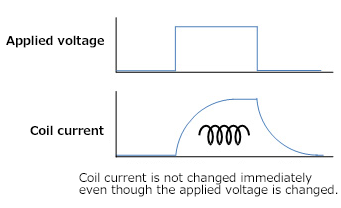A motor coil has the property to suppress changes in the flowing current.
The winding of a stepping motor is also made of coil, so the rated-current drive utilizes this property.

#### 2. Constant current control method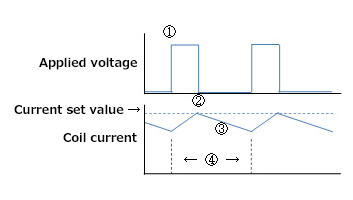Mostly, the constant-current controls with constant current driver ICs operate as follows:
(1) Apply voltage from the power supply to the coil
(2) Stop applying voltage from the power supply when the coil current increases and reaches the current set value.
(3) The energy stored in the coil is consumed by commutating the current through the path which can pass. At this time, the current flowing through the coil decays because the energy is consumed.
(4) Repeats the phases (1), (2), and (3) above at regular intervals to perform the constant-current control in which constant current flows.

#### 3. What is “decay control”?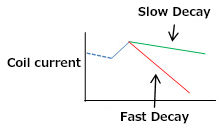In (3) above, we say “The energy stored in the coil is consumed by commutating the current through the path which can pass. By controlling this path, you can change how the current is decayed.

#### Fast Decay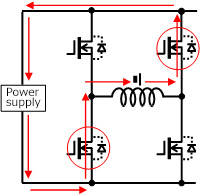The energy stored in the coil retains an electromotive force like a battery, and it is commutated (regenerated) via the power supply by turning ON the FETs [red circles in the figure] on the path flowing toward the power supply.
At this time, impedance of the power supply is low, so it decays quickly. This is called “Fast Decay”.
Note that when current flows through a parasitic diode, the forward voltage is lost, so the FET should be turned ON without using a diode.

#### Slow Decay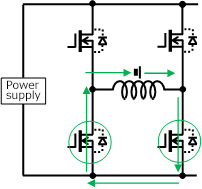Then, the coil can consume energy itself by turning ON the FETs [green circles in the figure] on the path commutating to a coil itself without passing through the power supply. (In some cases, the upper two FETs are turned ON.)
At this time, the impedance of a coil is relatively higher than that of the power supply, so it decays slowly. This is called “Slow Decay”.

### 4. When you use “Decay controls”?

Normally, Slow Decay, which consumes energy by the coil itself, is more efficient due to less current ripple. However, back EMF, which cannot be consumed, may be generated by the capacity of the motor used, the load, the speed, and the driving method (micro-step). This can lead to unintentional and reactive currents.

If Fast Decay is used in such a case, back EMF is absorbed by the power supply, so that reactive current flow can be prevented.
In this way, conducting Decay controls may be getting close to the ideal control current.

It looks somewhat difficult to use Decay controls since it is necessary to check the current waveform to determine whether or not Decay controls are required. However, there are some ICs that automatically perform Decay controls, and they have become effective functions for fine-tuning the response of the equipment.